Tamil Nadu Board of Secondary EducationSSLC (English Medium) Class 7th

# Tamil Nadu Board Samacheer Kalvi solutions for Class 7th Mathematics Term 2 Answers Guide chapter 4 - Geometry [Latest edition]

#### Chapters## Chapter 4: Geometry

Exercise 4.1Exercise 4.2Exercise 4.3
Exercise 4.1 [Pages 73 - 75]

### Tamil Nadu Board Samacheer Kalvi solutions for Class 7th Mathematics Term 2 Answers Guide Chapter 4 Geometry Exercise 4.1 [Pages 73 - 75]

Exercise 4.1 | Q 1 | Page 73

Can 30°, 60° and 90° be the angles of a triangle?

Exercise 4.1 | Q 2 | Page 73

Can you draw a triangle with 25°, 65° and 80° as angles?

Exercise 4.1 | Q 3. (i) | Page 73

In the following triangle, find the value of x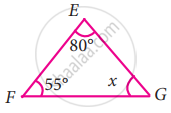Exercise 4.1 | Q 3. (ii) | Page 73

In the following triangle, find the value of x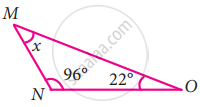Exercise 4.1 | Q 3. (iii) | Page 73

In the following triangle, find the value of x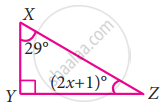Exercise 4.1 | Q 3. (iv) | Page 73

In the following triangle, find the value of x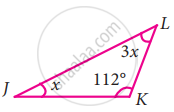Exercise 4.1 | Q 3. (v) | Page 73

In the following triangle, find the value of x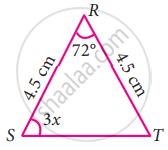Exercise 4.1 | Q 3. (vi) | Page 73

In the following triangle, find the value of x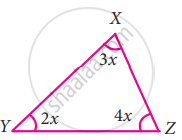Exercise 4.1 | Q 3. (vii) | Page 73

In the following triangle, find the value of x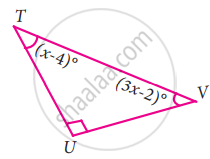Exercise 4.1 | Q 3. (viii) | Page 73

In the following triangle, find the value of x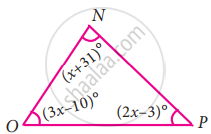Exercise 4.1 | Q 4 | Page 73

Two line segments bar("AD") and bar("BC") intersect at O. Joining bar("AB") and bar("DC") we get two triangles, ∆AOB and ∆DOC as shown in the figure. Find the ∠A and ∠B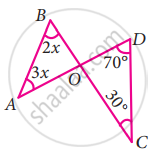Exercise 4.1 | Q 5 | Page 73

Observe the figure and find the value of ∠A + ∠N + ∠G + ∠L + ∠E + ∠S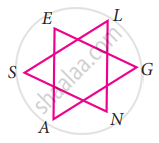Exercise 4.1 | Q 6 | Page 73

If the three angles of a triangle are in the ratio 3 : 5 : 4, then find them

Exercise 4.1 | Q 7 | Page 73

In ∆RST, ∠S is 10° greater than ∠R and ∠T is 5° less than ∠S, find the three angles of the triangle

Exercise 4.1 | Q 8 | Page 73

In ∆ABC, if ∠B is 3 times ∠A and ∠C is 2 times ∠A, then find the angle

Exercise 4.1 | Q 9 | Page 73

In ∆XYZ, if ∠X : ∠Z is 5 : 4 and ∠Y = 72°. Find ∠X and ∠Z

Exercise 4.1 | Q 10 | Page 74

In a right angled triangle ABC, ∠B is right angle, ∠A is x + 1 and ∠C is 2x + 5. Find ∠A and ∠C

Exercise 4.1 | Q 11 | Page 74

In a right angled triangle MNO, ∠N = 90°, MO is extended to P. If ∠NOP = 128°, find the other two angles of ∆MNO

Exercise 4.1 | Q 12. (i) | Page 74

Find the value of x in the given triangle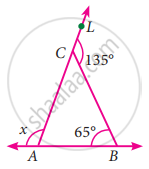Exercise 4.1 | Q 12. (ii) | Page 74

Find the value of x in the given triangle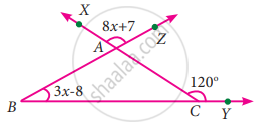Exercise 4.1 | Q 13 | Page 74

In ∆LMN, MN is extended to O. If ∠MLN = 100 – x, ∠LMN = 2x and ∠LNO = 6x – 5, find the value of x

Exercise 4.1 | Q 14 | Page 74

Using the given figure find the value of x.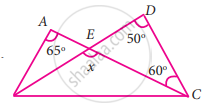Exercise 4.1 | Q 15 | Page 74

Using the diagram find the value of x.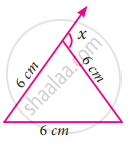#### Objective type questions

Exercise 4.1 | Q 16 | Page 74

The angles of a triangle are in the ratio 2 : 3 : 4. Then the angles are

• 20, 30, 40

• 40, 60, 80

• 80, 20, 80

• 10, 15, 20

Exercise 4.1 | Q 17 | Page 74

One of the angles of a triangle is 65°. If the difference of the other two angles is 45°, then the two angles are

• 85°, 40°

• 70°, 25°

• 80°, 35°

• 80°, 135°

Exercise 4.1 | Q 18 | Page 74

In the given figure, AB is parallel to CD. Then the value of b is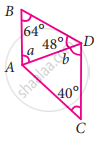• 112°

• 68°

• 102°

• 62°

Exercise 4.1 | Q 19 | Page 74

In the given figure, which of the following statement is true?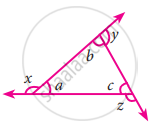• x + y + z = 180°

• x + y + z = a + b + c

• x + y + z = 2(a + b + c)

• x + y + z = 3(a + b + c)

Exercise 4.1 | Q 20 | Page 75

An exterior angle of a triangle is 70° and two interior opposite angles are equal. Then measure of each of these angle will be

• 110°

• 120°

• 35°

• 60°

Exercise 4.1 | Q 21 | Page 75

In a ∆ABC, AB = AC. The value of x is ________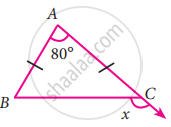• 80°

• 100°

• 130°

• 120°

Exercise 4.1 | Q 22 | Page 75

If an exterior angle of a triangle is 115° and one of the interior opposite angles is 35°, then the other two angles of the triangle are

• 45°, 60°

• 65°, 80°

• 65°, 70°

• 115°, 60°

Exercise 4.2 [Pages 83 - 86]

### Tamil Nadu Board Samacheer Kalvi solutions for Class 7th Mathematics Term 2 Answers Guide Chapter 4 Geometry Exercise 4.2 [Pages 83 - 86]

Exercise 4.2 | Q 1. (i) | Page 83

Given that ∆ABC ≅ ∆DEF List all the corresponding congruent sides

Exercise 4.2 | Q 1. (ii) | Page 83

Given that ∆ABC ≅ ∆DEF List all the corresponding congruent angles

Exercise 4.2 | Q 2. (i) | Page 83

If the given two triangles are congruent, then identify all the corresponding sides and also write the congruent angles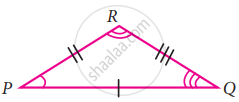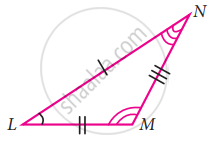Exercise 4.2 | Q 2. (ii) | Page 84

If the given two triangles are congruent, then identify all the corresponding sides and also write the congruent angles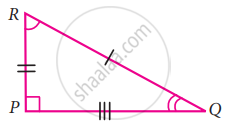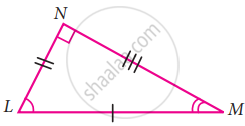Exercise 4.2 | Q 3. (i) | Page 84

If the given triangles ΔABC and ΔEFG are congruent, determine whether the given pair of sides and angles are corresponding sides or corresponding angles or not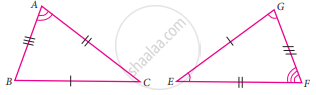∠A and ∠G

Exercise 4.2 | Q 3. (ii) | Page 84

If the given triangles ΔABC and ΔEFG are congruent, determine whether the given pair of sides and angles are corresponding sides or corresponding angles or not∠B and ∠E

Exercise 4.2 | Q 3. (iii) | Page 84

If the given triangles ΔABC and ΔEFG are congruent, determine whether the given pair of sides and angles are corresponding sides or corresponding angles or not∠B and ∠G

Exercise 4.2 | Q 3. (iv) | Page 84

If the given triangles ΔABC and ΔEFG are congruent, determine whether the given pair of sides and angles are corresponding sides or corresponding angles or notbar("AC") and bar("GF")

Exercise 4.2 | Q 3. (v) | Page 84

If the given triangles ΔABC and ΔEFG are congruent, determine whether the given pair of sides and angles are corresponding sides or corresponding angles or notbar("BA") and bar("FG")

Exercise 4.2 | Q 3. (vi) | Page 84

If the given triangles ΔABC and ΔEFG are congruent, determine whether the given pair of sides and angles are corresponding sides or corresponding angles or notbar("EF") and bar("BC")

Exercise 4.2 | Q 4. (i) | Page 84

State whether the two triangles are congruent or not. Justify your answer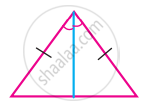Exercise 4.2 | Q 4. (ii) | Page 84

State whether the two triangles are congruent or not. Justify your answer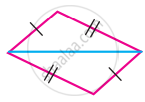Exercise 4.2 | Q 4. (iii) | Page 84

State whether the two triangles are congruent or not. Justify your answer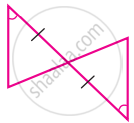Exercise 4.2 | Q 4. (iv) | Page 84

State whether the two triangles are congruent or not. Justify your answer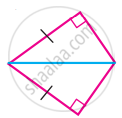Exercise 4.2 | Q 4. (v) | Page 84

State whether the two triangles are congruent or not. Justify your answer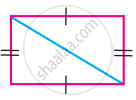Exercise 4.2 | Q 5. (i) | Page 84

To conclude the congruency of triangles, mark the required information in the following figure with reference to the given congruency criterion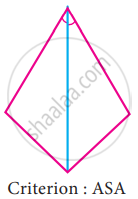Exercise 4.2 | Q 5. (ii) | Page 84

To conclude the congruency of triangles, mark the required information in the following figure with reference to the given congruency criterion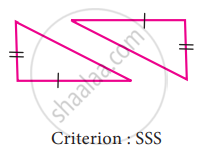Exercise 4.2 | Q 5. (iii) | Page 84

To conclude the congruency of triangles, mark the required information in the following figure with reference to the given congruency criterion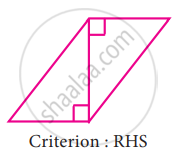Exercise 4.2 | Q 5. (iv) | Page 84

To conclude the congruency of triangles, mark the required information in the following figure with reference to the given congruency criterion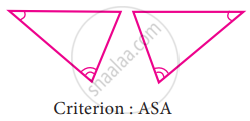Exercise 4.2 | Q 5. (v) | Page 84

To conclude the congruency of triangles, mark the required information in the following figure with reference to the given congruency criterion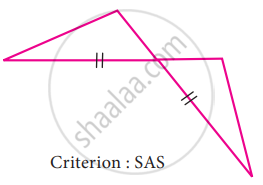Exercise 4.2 | Q 6. (i) | Page 85

For the given pair of triangles state the criterion that can be used to determine the congruency?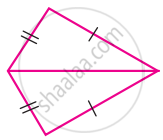Exercise 4.2 | Q 6. (ii) | Page 85

For the given pair of triangles state the criterion that can be used to determine the congruency?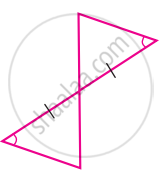Exercise 4.2 | Q 6. (iii) | Page 85

For the given pair of triangles state the criterion that can be used to determine the congruency?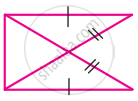Exercise 4.2 | Q 6. (iv) | Page 85

For the given pair of triangles state the criterion that can be used to determine the congruency?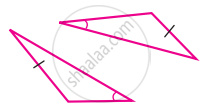Exercise 4.2 | Q 6. (v) | Page 85

For the given pair of triangles state the criterion that can be used to determine the congruency?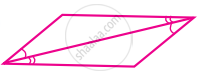Exercise 4.2 | Q 6. (vi) | Page 85

For the given pair of triangles state the criterion that can be used to determine the congruency?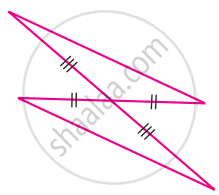Exercise 4.2 | Q 7. I. (i) | Page 85

Construct a triangle XYZ with the given conditions.

XY = 6.4 cm, ZY = 7.7 cm and XZ = 5 cm

Exercise 4.2 | Q 7. I. (ii) | Page 85

Construct a triangle XYZ with the given conditions.

An equilateral triangle of side 7.5 cm

Exercise 4.2 | Q 7. I. (iii) | Page 85

Construct a triangle XYZ with the given conditions.

An isosceles triangle with equal sides 4.6 cm and third side 6.5 cm

Exercise 4.2 | Q 7. II. (i) | Page 85

Construct a triangle ABC with given conditions.

AB = 7 cm, AC = 6.5 cm and ∠A = 120°

Exercise 4.2 | Q 7. II. (ii) | Page 85

Construct a triangle XYZ with the given conditions.

BC = 8 cm, AC = 6 cm and ∠C = 40°

Exercise 4.2 | Q 7. II. (iii) | Page 85

Construct a triangle XYZ with the given conditions.

An isosceles obtuse triangle with equal sides 5 cm

Exercise 4.2 | Q 7. III. (i) | Page 85

Construct a triangle PQR with given conditions.

∠P = 60°, ∠R = 35° and PR = 7.8 cm

Exercise 4.2 | Q 7. III. (ii) | Page 85

Construct a triangle PQR with given conditions.

∠P = 115°, ∠Q = 40° and PQ = 6 cm

Exercise 4.2 | Q 7. III. (iii) | Page 85

Construct a triangle PQR with given conditions.

∠Q = 90°, ∠R = 42° and QR = 5.5 cm

#### Objective type questions

Exercise 4.2 | Q 8 | Page 85

If two plane figures are congruent then they have

• same size

• same shape

• same angle

• same shape and same size

Exercise 4.2 | Q 9 | Page 85

Which of the following methods are used to check the congruence of plane figures?

• translation method

• superposition method

• substitution method

• transposition method

Exercise 4.2 | Q 10 | Page 85

Which of the following rule is not sufficient to verify the congruency of two triangles

• SSS rule

• SAS rule

• SSA rule

• ASA rule

Exercise 4.2 | Q 11 | Page 86

Two students drew a line segment each. What is the condition for them to be congruent?

• They should be drawn with a scale

• They should be drawn on the same sheet of paper

• They should have different lengths

• They should have the same length

Exercise 4.2 | Q 12 | Page 86

In the given figure, AD = CD and AB = CB. Identify the other three pairs that are equal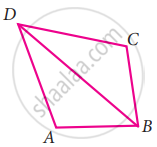• ∠ADB = ∠CDB, ∠ABD = ∠CBD, BD = BD

• AD = AB, DC = CB, BD = BD

• AB = CD, AD = BC, BD = BD

• ∠ADB = ∠CDB, ∠ABD = ∠CBD, ∠DAB = ∠DBC

Exercise 4.2 | Q 13 | Page 86

In ∆ABC and ∆PQR, ∠A = 50° = ∠P, PQ = AB, and PR = AC. By which property ∆ABC and ∆PQR are congruent?

• SSS property

• SAS property

• ASA property

• RHS property

Exercise 4.3 [Pages 86 - 87]

### Tamil Nadu Board Samacheer Kalvi solutions for Class 7th Mathematics Term 2 Answers Guide Chapter 4 Geometry Exercise 4.3 [Pages 86 - 87]

#### Miscellaneous Practice problems

Exercise 4.3 | Q 1 | Page 86

In an isosceles triangle one angle is 76°. If the other two angles are equal find them

Exercise 4.3 | Q 2 | Page 86

If two angles of a triangle are 46° each, how can you classify the triangle?

Exercise 4.3 | Q 3 | Page 86

If an angle of a triangle is equal to the sum of the other two angles, find the type of the triangle

Exercise 4.3 | Q 4 | Page 86

If the exterior angle of a triangle is 140° and its interior opposite angles are equal, find all the interior angles of the triangle

Exercise 4.3 | Q 5 | Page 86

In ∆JKL, if ∠J = 60° and ∠K = 40°, then find the value of exterior angle formed by extending the side KL

Exercise 4.3 | Q 6 | Page 86

Find the value of ‘x’ in the given figure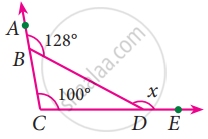Exercise 4.3 | Q 7 | Page 86

If ∆MNO ≅ ∆DEF, ∠M = 60° and ∠E = 45° then find the value of ∠O

Exercise 4.3 | Q 8. (i) | Page 86

In the given figure ray AZ bisects ∠BAD and ∠DCB, prove that ∆BAC ≅ ∆DAC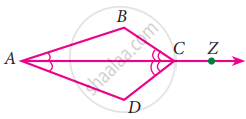Exercise 4.3 | Q 8. (ii) | Page 86

In the given figure ray AZ bisects ∠BAD and ∠DCB, prove that AB = ADExercise 4.3 | Q 9 | Page 87

In the given figure FG = FI and H is midpoint of GI, prove that ∆FGH ≅ ∆FHI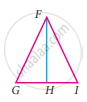Exercise 4.3 | Q 10 | Page 87

Using the given figure, prove that the triangles are congruent. Can you conclude that AC is parallel to DE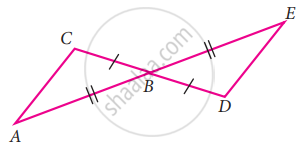#### Challenge Problems

Exercise 4.3 | Q 11 | Page 87

In the given figure BD = BC, find the value of x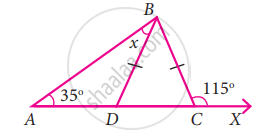Exercise 4.3 | Q 12 | Page 87

In the given figure find the value of x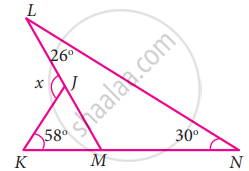Exercise 4.3 | Q 13 | Page 87

In the given figure find the values of x and y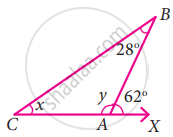Exercise 4.3 | Q 14 | Page 87

In ∆DEF, ∠F = 48°, ∠E = 68° and bisector of ∠D meets FE at G. Find ∠FGD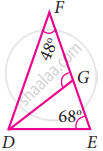Exercise 4.3 | Q 15 | Page 87

In the figure find the value of x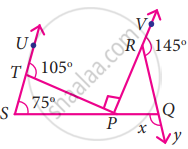Exercise 4.3 | Q 16 | Page 87

From the given figure find the value of y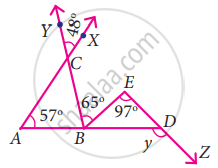## Chapter 4: Geometry

Exercise 4.1Exercise 4.2Exercise 4.3## Tamil Nadu Board Samacheer Kalvi solutions for Class 7th Mathematics Term 2 Answers Guide chapter 4 - Geometry

Tamil Nadu Board Samacheer Kalvi solutions for Class 7th Mathematics Term 2 Answers Guide chapter 4 (Geometry) include all questions with solution and detail explanation. This will clear students doubts about any question and improve application skills while preparing for board exams. The detailed, step-by-step solutions will help you understand the concepts better and clear your confusions, if any. Shaalaa.com has the Tamil Nadu Board of Secondary Education Class 7th Mathematics Term 2 Answers Guide solutions in a manner that help students grasp basic concepts better and faster.

Further, we at Shaalaa.com provide such solutions so that students can prepare for written exams. Tamil Nadu Board Samacheer Kalvi textbook solutions can be a core help for self-study and acts as a perfect self-help guidance for students.

Concepts covered in Class 7th Mathematics Term 2 Answers Guide chapter 4 Geometry are Concept of Triangles - Sides, Angles, Vertices, Interior and Exterior of Triangle, Classification of Triangles (On the Basis of Sides, and of Angles), Equilateral Triangle, Isosceles Triangles, Scalene Triangle, Acute Angled Triangle, Exterior Angle of a Triangle and Its Property, Congruence Among Line Segments, Congruence of Plane Figures, Congruence of Angles, Congruence of Triangles, SSS Congruence Criterion, SAS Congruence Criterion, ASA Congruence Criterion, RHS Congruence Criterion, Obtuse Angled Triangle, Right Angled Triangle, Angle Sum Property of a Triangle, Congruence of Triangles, Congruency of Shapes, Criteria for Congruence of Triangles.

Using Tamil Nadu Board Samacheer Kalvi Class 7th solutions Geometry exercise by students are an easy way to prepare for the exams, as they involve solutions arranged chapter-wise also page wise. The questions involved in Tamil Nadu Board Samacheer Kalvi Solutions are important questions that can be asked in the final exam. Maximum students of Tamil Nadu Board of Secondary Education Class 7th prefer Tamil Nadu Board Samacheer Kalvi Textbook Solutions to score more in exam.

Get the free view of chapter 4 Geometry Class 7th extra questions for Class 7th Mathematics Term 2 Answers Guide and can use Shaalaa.com to keep it handy for your exam preparation